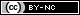# How could the different inventory costing methods used by Best Buy, Amazon, and Target impact their respective income statements and balance sheets?Three retailing giants, Best Buy Co., Inc. (NYSE: BBY), Amazon.com, Inc., (NASDAQ: AMZN), and Target Corporation (NYSE: TGT) each use a different inventory costing method. Best Buy uses weighted-average cost, Amazon uses FIFO, and Target uses LIFO.

Here is a hypothetical example that highlights the potential differences in income statements and balance sheets that could arise simply because of the use of a different inventory costing method.

Basic facts

Assume that all three retailers sell a popular shirt that retails for \$32. To compare the impact of inventory costing method, we will also assume that all three retailers have the following inventory and sales data for the same period. To keep the calculations simple, a “unit” represents one million shirts.

• Beginning inventory: 3 units @ \$10.00
• Purchases: 2 units @ \$15.00
• Ending inventory: 1 unit

Questions

1. Calculate the number of units sold.
2. Calculate cost of goods sold, cost of ending inventory, and gross margin for Best Buy using the weighted-average inventory costing method.
3. Calculate cost of goods sold, cost of ending inventory, and gross margin for Amazon using the FIFO inventory costing method.
4. Calculate cost of goods sold, cost of ending inventory, and gross margin for Target using the LIFO inventory costing method.
5. If prices are decreasing, would FIFO or LIFO produce the highest income? Explain.
6. Would FIFO or LIFO produce the highest inventory cost on the balance sheet if prices are rising? Explain.

Instructor Resources

These resources are provided to give the instructor flexibility for use of Accounting in the Headlines articles in the classroom. The blog posting itself can be assigned via a link to this site OR by distributing the student handout below. Alternatively, the PowerPoint file below contains a bullet point overview of the article and the discussion questions.

• Student handout (pdf) (word) (contains entire blog posting + discussion questions)
• PowerPoint file (brief article overview + discussion questions + calculations for solution)
• Excel solutions file (includes calculations)